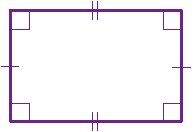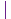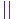# Rectangle

A rectangle is a four-sided flat shape where every angle is a right angle (90°).means "right angle"are equal sidesare equal sidesEach internal angle is 90°Opposite sides are parallel and of equal length (so it is a Parallelogram).

Play with a rectangle:

## Area of a RectangleArea = a × b

Area= 6 m × 3 m
= 18 m2

## Perimeter of a Rectangle

The Perimeter is the distance around the edges.The Perimeter is 2 times (a + b): Perimeter = 2(a+b)

### Example: A rectangle is 12 cm long and 5 cm tall, what is its Perimeter?

Perimeter = 2 × (12 cm + 5 cm)
= 2 × 17 cm
= 34 cm

## Diagonals of a RectangleA rectangle has two diagonals, they are equal in length and intersect in the middle.A diagonal's length is the square root of (a squared + b squared): Diagonal "d" = √(a2 + b2)

### Example: A rectangle is 12 cm wide, and 5 cm tall, what is the length of a diagonal?d = √(122 + 52)
= √(144 + 25)
= √169
= 13 cm## Golden Rectangle

There is also a special rectangle called the Golden Rectangle: